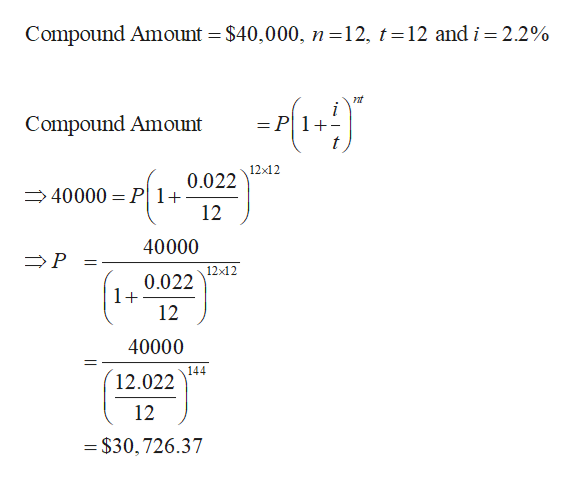# Use the present value formula to determine the amount to be invested​ now, or the present value neededThe desired accumulated amount is \$40,000 after 12 years invested in an account with 2.2​% interest compounded monthly.The amount to be invested​ now, or the present value​ needed, is ​\$__Round to the nearest cent as needed

Question
1 views

Use the present value formula to determine the amount to be invested​ now, or the present value needed

The desired accumulated amount is \$40,000 after
12 years invested in an account with 2.2​% interest compounded monthly.

The amount to be invested​ now, or the present value​ needed, is ​\$__

Round to the nearest cent as needed

check_circle

Step 1

Recall the following fact.help_outlineImage Transcriptionclosent Compound Amount = P1+ where P is the Principal amount i is the annual interest rate n is the number of years t is the number of times compounded per year fullscreen
Step 2

Substitute the given values and compute the co...help_outlineImage TranscriptioncloseCompound Amount = \$40,000, n =12, t = 12 and i = 2.2% nt = P Compound Amount 12x12 0.022 40000 P 1 12 40000 P 12x12 0.022 1+ 12 40000 144 12.022 12 = \$30,726.37 fullscreen

### Want to see the full answer?

See Solution

#### Want to see this answer and more?

Solutions are written by subject experts who are available 24/7. Questions are typically answered within 1 hour.*

See Solution
*Response times may vary by subject and question.
Tagged in

### Math